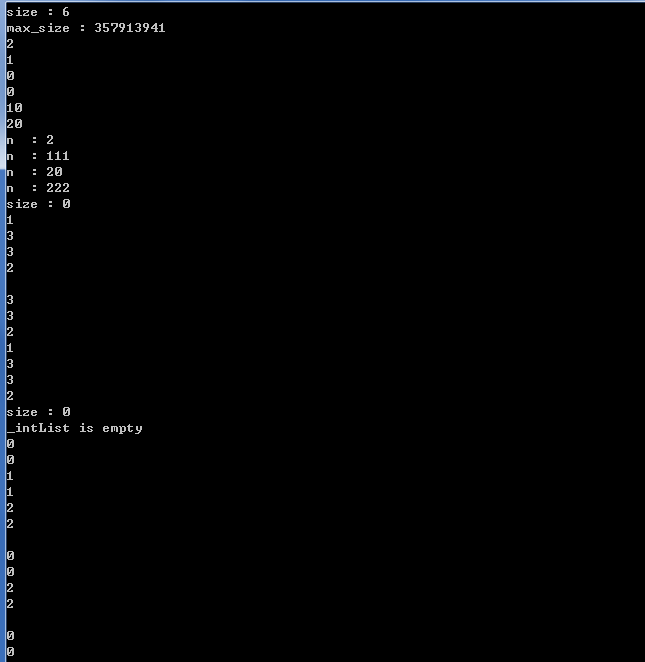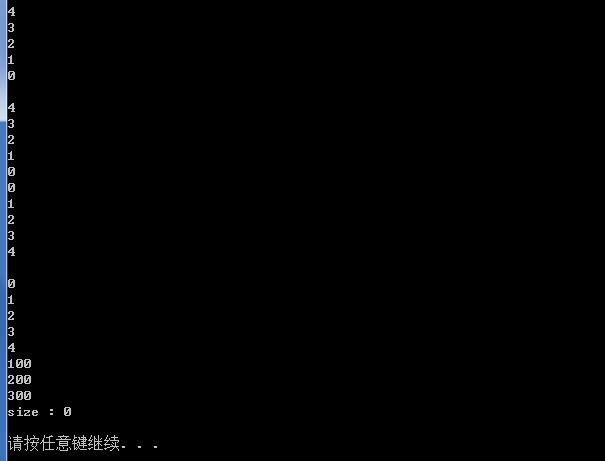• ## c++list

千次阅读 2019-06-14 14:01:37
c++ list头文件 简介 list实际上是双向链表，故亦可称之为doubly-linked list 性质 双向 链表 双向 双向即给定一个元素，我们能够知道后一个元素和前一个元素 list的迭代器是双向迭代器 链表...
c++ list头文件
简介
双向链表
双向
双向即给定一个元素，我们能够知道后一个元素和前一个元素list的迭代器是双向迭代器
链表
优点：与向量(vectors)相比，它可以快速的插入和删除–插入和删除操作是常数时间的缺点：随机访问比较慢–元素的访问不是常数时间的，获取元素往往需要在给定一个迭代器的基础上来通过遍历实现时间复杂度
常数时间O(1)(与输入数据无关): 基本操作重复执行的次数是一个固定的常数，执行次数不存在变化，通俗的讲就是：无论n是什么值运算所花时间都一样线性时间O(n)(与输入数据成正比): 基本操作重复执行的次数是与模块n成线性相关的，其值会随着模块n的变化而变化，当模块n的规模确定为定值后，其时间复杂度转化为O(1)
List
定义和初始化
listlst1; // 创建空listlistlst2(6); //创建含有6个元素的listlistlst3(3, 2); // 创建含有三个元素的listlistlst4(lst2); // 使用ls2初始化ls4listlst5(lst2.begin(), lst2.end()); // 同ls4
list常用操作函数
lst1.assign() // 给list赋值lst1.front() // 返回第一个元素lst1.back() // 返回最后一个元素lst1.begin() // 返回指向第一个元素的迭代器lst1.end() // 返回末尾的迭代器lst1.insert() // 插入一个元素到list中lst1.erase() // 删除一个元素lst1.pop_back() // 删除最有一个元素lst1.pop_front() // 删除第一个元素lst1.clear() // 删除所有元素lst1.remove(const T & val) // 删除和val相等的元素lst1.push_back() // 在list的末尾添加一个元素lst1.push_front() // 在list的首部添加一个元素lst1.empty() // 判断，若list为空返回truelst1.max_size() // 返回list能容纳的最大元素数量lst1.sort() // 给list排序(顺序)list.reverse() // 把list中的元素倒转lst1.merge(lst2) // 合并lst2到lst1，并清空lst2lst1.unique() // 删除所有和前一个元素相等的元素void splice(iterator i, list & x, iterator first, iterator last) // 在位置i前面插入链表x中的区间 [first, last）, 并在链表x中删除该区间（链表自身和链表x可以是用一个链表，只要i不在 [first, last) 中即可
list案例
#include <list>  // 使用 list 需要包含此头文件
#include <algorithm>  // 使用 STL 中的算法需要包含此头文件
#include <iostream>
using namespace std;

class A
{
public:
A(int n_):n(n_){}
friend bool operator < (const A & a1, const A & a2);
friend bool operator == (const A & a1, const A & a2);
friend ostream & operator << (ostream & out, const A & a);
private:
int n;
};

bool operator < (const A & a1, const A & a2){
return a1.n < a2.n;
}

bool operator == (const A & a1, const A & a2){
return a1.n == a2.n;
}

ostream & operator << (ostream & out, const A & a){
out << a.n;
return out;
}
template <class T>
void Print(T first, T last)
{
for(; first != last; ++first)
cout<<*first<<" ";
cout<<endl;
}

int main()
{
A a = {1, 3, 2, 4, 2};
A b = {10, 30, 20, 30, 30, 40, 40};
list<A> lst1(a, a+5), lst2(b, b+7);
lst1.sort();    // 顺序排序
cout<<"1. "; Print(lst1.begin(), lst1.end());
lst1.remove(2);  // 删除所有和A(2)相等的元素
cout<<"2. "; Print(lst1.begin(), lst1.end());
lst2.pop_front();  // 删除第一个元素
cout<<"3. "; Print(lst2.begin(), lst2.end());
lst2.unique();  // 删除所有和前一个元素相等的元素
cout<<"4. "; Print(lst2.begin(), lst2.end());
lst2.sort();  // 顺序排序
lst1.merge(lst2);  // 合并 lst2 到 lst1 并清空 lst2
cout<<"5. "; Print(lst1.begin(), lst1.end());
cout<<"6. "; Print(lst2.begin(), lst2.end());  // lst2 是空的
lst1.reverse();  // 将 lst1 倒置

cout<<"7. "; Print(lst1.begin(), lst1.end());
lst2.insert(lst2.begin(), a + 1, a + 4);  // 在 lst2 中插入 3，2，4 三个元素
list<A>::iterator p1, p2, p3;
p1 = find(lst1.begin(), lst1.end(), 30);  // 查找元素
p2 = find(lst2.begin(), lst2.end(), 2);
p3 = find(lst2.begin(), lst2.end(), 4);
lst1.splice(p1, lst2, p2, p3);  // 将 [p2, p3) 插入p1之前，并从lst2中删除 [p2, p3)
cout<<"8. "; Print(lst1.begin(), lst1.end());
cout<<"9. "; Print(lst2.begin(), lst2.end());
return 0;
}

1. 1 2 2 3 4
2. 1 3 4
3. 30 20 30 30 40 40
4. 30 20 30 40
5. 1 3 4 20 30 30 40
6.
7. 40 30 30 20 4 3 1
8. 40 2 30 30 20 4 3 1
9. 3 4
请按任意键继续. . .

【实例】用 list 解决约瑟夫问题
约瑟夫问题是：有 n 只猴子，按顺时针方向围成一圈选大王（编号为 1~n），从第 1 号开始报数，一直数到 m，数到 m 的猴子退到圈外，剩下的猴子再接着从 1 开始报数。就这样，直到圈内只剩下一只猴子时，这个猴子就是猴王。编程求输入 n、m 后,输出最后猴王的编号。
输入数据：每行是用空格分开的两个整数，第一个是 n，第二个是 m（0<m, n<=1 000 000）。最后一行是： 0 0
输出要求：对于每行输入数据（最后一行除外），输出数据也是一行，即最后猴王的编号。
输入样例： 6 2 12 4 8 3 0 0
输出样例： 5 1 7
程序如下
#include <list>
#include <iostream>
using namespace std;

int main()
{
list<int> monkeys;
int n, m;
while(1)
{
cin >> n >> m;
if(n == 0 && m == 0)
break;
monkeys.clear();  // 清空list容器
for(int i = 0; i <= n; ++ i)  // 将猴子的编号放入list中
monkeys.push_back(i);
list<int>::iterator it = monkeys.begin();  // 定义一个迭代器
while(monkeys.size() > 1)  // 只要还有不止一只猴子，就要找一只猴子让其出列
{
for(int i = 1; i < m; ++ i)  // 报数
{
++it;
if(it == monkeys.end())
it = monkeys.begin();
}
it = monkeys.erase(it);  // 删除元素后，迭代器失效
// 要重新让迭代器指向被删元素的后面
if(it == monkeys.end())
it = monkeys.begin();
}
cout<<monkeys.front()<<endl;  // front返回第一个元素的引用
}
return 0;
}

10000 3
2694
0 0
请按任意键继续. . .

erase 成员函数返回被删除元素后面那个元素的迭代器，如果被删除的是最后一个元素，则返回end()
展开全文• ## C++list

千次阅读 2010-11-09 15:08:00
Source:#include #include ... //创建一个list容器的实例LISTINT typedef list LISTCHAR; //创建一个list容器的实例LISTCHAR int main(void) { LISTINT listOne; //用LISTINT创建一个名为listOne的l

Source:
#include <iostream>
#include <list>
#include <numeric>
#include <algorithm>
using namespace std;

typedef list<int> LISTINT;   //创建一个list容器的实例LISTINT
typedef list<int> LISTCHAR;  //创建一个list容器的实例LISTCHAR
int main(void)  {
LISTINT listOne;    //用LISTINT创建一个名为listOne的list对象
LISTINT::iterator i;    //声明i为迭代器
listOne.push_front (2); //从前面向listOne容器中添加数据
listOne.push_front (1);
listOne.push_back (3); 	//从后面向listOne容器中添加数据
listOne.push_back (4);

cout<<"listOne.begin()--- listOne.end():"<<endl;   //从前向后显示listOne中的数据
for (i = listOne.begin(); i != listOne.end(); ++i)
cout << *i << " ";
cout << endl;

LISTINT::reverse_iterator ir;    //从后向后显示listOne中的数据
cout<<"listOne.rbegin()---listOne.rend():"<<endl;
for (ir =listOne.rbegin(); ir!=listOne.rend();ir++)
cout << *ir << " ";
cout << endl;

int result = accumulate(listOne.begin(), listOne.end(),0);  //使用STL的accumulate(累加)算法
cout<<"Sum="<<result<<endl;

LISTCHAR listTwo;   //用LISTCHAR创建一个名为listOne的list对象
LISTCHAR::iterator j;      //声明j为迭代器
listTwo.push_front ('A');  //从前面向listTwo容器中添加数据
listTwo.push_front ('B');
listTwo.push_back ('x');   //从后面向listTwo容器中添加数据
listTwo.push_back ('y');
cout<<"listTwo.begin()---listTwo.end():"<<endl; //从前向后显示listTwo中的数据
for (j = listTwo.begin(); j != listTwo.end(); ++j)
cout << char(*j) << " ";
cout << endl;
//使用STL的max_element算法求listTwo中的最大元素并显示
j=max_element(listTwo.begin(),listTwo.end());
cout << "The maximum element in listTwo is: "<<char(*j)<<endl;
return 0;
}

Result:

[work@db-testing-com06-vm3.db01.baidu.com c++]$g++ -o list list.cpp [work@db-testing-com06-vm3.db01.baidu.com c++]$ ./list
listOne.begin()--- listOne.end():
1 2 3 4
listOne.rbegin()---listOne.rend():
4 3 2 1
Sum=10
listTwo.begin()---listTwo.end():
B A x y
The maximum element in listTwo is: y


展开全文iterator iostream algorithm
• 主要介绍了 C++ list的实例详解的相关资料,希望通过本文大家能够理解掌握这部分内容，需要的朋友可以参考下
• C++的学习资料很有用 适合初学者 C++ List学习 有例子
• C++编程，ListControl控件应用，C++控件进阶应用，程序实例
• c++ listClass 介绍，某大学课件，英文
• ## C++list 使用方法

千次阅读 2016-01-24 14:24:17
C++ list 使用方法使用 list需引入头文件#include <list>引入命名空间 using namespace std;list函数如下//assign() 给list赋值 //back() 返回最后一个元素的引用 //begin() 返回指向容器第一个元素的指针 //clear()...
C++ list 使用方法
使用 list需引入头文件#include <list>
引入命名空间 using namespace std;
list函数如下
//assign()      给list赋值
//back()        返回最后一个元素的引用
//begin()       返回指向容器第一个元素的指针
//clear()       删除所有元素
//empty()       如果list是空的则返回true
//end()         返回容器末尾的指针
//erase()       删除一个元素
//front()       返回第一个元素
//get_allocator() 返回list的配置器
//insert()      插入一个元素到容器中
//max_size()    返回list能容纳的最大元素数量
//merge()       合并两个list
//pop_back()    删除最后一个元素
//pop_front()   删除第一个元素
//push_back()   在list的末尾添加一个元素
//push_front()  在list的头部添加一个元素
//rbegin()      返回指向第一个元素的逆向迭代器
//remove()      从list删除元素
//remove_if()   按指定条件删除元素
//rend()        指向list末尾的逆向迭代器
//resize()      改变list的大小
//reverse()     把list的元素倒转
//size()        返回list中的元素个数
//sort()        给list排序
//splice()      合并两个list
//swap()        交换两个list
//unique()      删除list中重复的元素
使用实例如下
// Pro1.cpp : 定义控制台应用程序的入口点。
//

#include "stdafx.h"

#include <list>

using namespace std;

// 创建一个 list 容器的实例 intList
typedef list<int> intList;

bool Is2(int i);

bool Sort(int i, int j);

int main()
{
intList _intList;

for (int i = 0; i < 3; ++i)
{
// 在容器头部添加一个元素
_intList.push_front(i);
}

for (int i = 0; i < 3; ++i)
{
// 在容器末尾添加一个元素
_intList.push_back(i * 10);
}

// 获取容器存储元素的个数
int size = _intList.size();
std::cout << "size : " << size <<endl;  // size : 6

// 获取容器可以存储的最大元素个数
int max_size = _intList.max_size();
std::cout << "max_size : " << max_size << endl;   // max_size : 357913941

// 声明 it 为容器迭代器
intList::iterator it;

// 获取指向容器第一个元素的指针
//_intList.begin()

// 获取指向容器最后一个元素 +1 的指针
// _intList.end()

// 使用迭代器遍历容器
for (it = _intList.begin(); it != _intList.end(); ++it)
{
std::cout << *it << endl;  // 2, 1, 0, 0, 10, 20
}

// front()获取容器第一个元素的引用
int n = _intList.front();
std::cout << "n  : "<< n << endl;  // n  : 2

// front() 作为第一个元素的引用，可以作为左值使用
_intList.front() = 111;
n = _intList.front();
std::cout << "n  : " << n << endl;  // n  : 111

// back() 返回最后一个元素的引用
n = _intList.back();
std::cout << "n  : " << n << endl;  // n  : 20

// back() 作为最后一个元素的引用，可以作为左值使用
_intList.back() = 222;
n = _intList.back();
std::cout << "n  : " << n << endl;  // n  : 222

// 使用迭代器遍历容器
it = _intList.begin();
for (; it != _intList.end();)
{
// 删除一个元素
_intList.erase(it++);
}

size = _intList.size();
std::cout << "size : " << size << endl;  // size : 0

// 在容器第一个元素位置插入数据 1,返回迭代器 it 位置处的指针
it = _intList.begin();
it = _intList.insert(it, 1);
++it;
// 在迭代器 it 位置插入数据 2
it = _intList.insert(it, 2);

// 在迭代器 it 位置插入 2 个数据 3
it = _intList.insert(it, 2, 3);

for (it = _intList.begin(); it != _intList.end(); ++it)
{
std::cout << *it << endl;   // 1, 3, 3, 2
}

std::cout << "" << endl;
//
it = _intList.begin();
// 在_intList.begin()位置开始，插入++it和_intList.end() 之间的数据
_intList.insert(_intList.begin(), ++it, _intList.end());

for (it = _intList.begin(); it != _intList.end(); ++it)
{
std::cout << *it << endl;   // 3, 3, 2, 1, 3, 3, 2
}

// 删除容器第一个元素
_intList.pop_front();
// 删除容器最后一个元素
_intList.pop_back();

// 删除容器所有元素
_intList.clear();
size = _intList.size();
std::cout << "size : " << size << endl;  // size : 0

// 判断容器是否为空
if (_intList.empty())
{
std::cout << "_intList is empty" << endl;
}

for (int i = 0; i < 3; i ++)
{
_intList.insert(it, i);
_intList.insert(it, i);
}

for (it = _intList.begin(); it != _intList.end(); ++it)
{
std::cout << *it << endl;   // 0, 0, 1, 1, 2, 2,
}

std::cout << "" << endl;   // 0, 0, 1, 1, 2, 2,

// 删除容器中所有值为 1 的元素
_intList.remove(1);
for (it = _intList.begin(); it != _intList.end(); ++it)
{
std::cout << *it << endl;   // 0, 0, 2, 2,
}

std::cout << "" << endl;

// 删除容器中符合 函数 Is2 返回 true 的值
_intList.remove_if(Is2);
for (it = _intList.begin(); it != _intList.end(); ++it)
{
std::cout << *it << endl;   // 0, 0,
}

std::cout << "" << endl;

// 改变容器存储空间大小（可以存储元素个数）
_intList.resize(10);
// 改变容器存储空间大小，新添加数据默认填充 100
_intList.resize(10, 100);

_intList.clear();

for (int i = 0; i < 5; i++)
{
_intList.push_back(i);
}

// 将容器中的数据反转
_intList.reverse();

for (it = _intList.begin(); it != _intList.end(); ++it)
{
std::cout << *it << endl;   // 4, 3, 2, 1, 0
}

std::cout << "" << endl;

_intList.push_back(0);

// 删除容器中重复的元素
_intList.unique();

for (it = _intList.begin(); it != _intList.end(); ++it)
{
std::cout << *it << endl;   // 4, 3, 2, 1, 0
}

// 将容器中数据按照 Sort 函数排序
_intList.sort(Sort);
for (it = _intList.begin(); it != _intList.end(); ++it)
{
std::cout << *it << endl;   // 4, 3, 2, 1, 0
}
std::cout << "" << endl;

intList _intList2;

_intList2.push_back(100);
_intList2.push_back(200);
_intList2.push_back(300);

// 将容器 _intList2容器合并到 _intList
_intList.merge(_intList2);
for (it = _intList.begin(); it != _intList.end(); ++it)
{
std::cout << *it << endl;   // 0, 1, 2, 3, 4, 100, 200, 300
}

// _intList2 数据已清空
size = _intList2.size();
std::cout << "size : "<< size << endl;   // size : 0

std::cout << "" << endl;

system("pause");
return 0;
}

bool Is2(int i)
{
return i == 2;
}

bool Sort(int i, int j)
{
return i < j;
}
运行结果展开全文容器 namespace
• 本篇文章是对C++ list中erase与remove函数的使用进行了详细的分析介绍，需要的朋友参考下
• c++ list 遍历中删除节点#include #include<list> using namespace std;int main() { int a = { 0, 1, 2, 3, 4, 5, 6, 7, 8, 9, 5, 10}; list<int> li(a, a + 12); //遍历删除值为5的节点 for (list<in
c++ list 遍历中删除节点
#include<iostream>
#include<list>
using namespace std;

int main()
{
int a = { 0, 1, 2, 3, 4, 5, 6, 7, 8, 9, 5, 10};
list<int> li(a, a + 12);
//遍历删除值为5的节点
for (list<int>::iterator it = li.begin(); it != li.end();)
{
if (*it == 5)
{
li.erase(it++); // 删除节点，并到下一个节点
}
else
{
++it;
}
}
for (list<int>::iterator it = li.begin(); it != li.end(); ++it)
{
cout << *it << " ";
}
system("pause");
}

展开全文链表
• C++ List的用法+遍历–学习总结 相关博客推荐： list用法详解 lis用法总结 list的遍历 下面提供两类list的遍历方法，一种是单纯list<>列表的遍历，另一种是l类似ist<list>这样的嵌套类型的list遍历 ...编程语言 列表
• ## c++list 正确删除

千次阅读 2019-01-05 15:25:40
c++ std::list 是一个双向链表，对它的遍历大部分都是使用list&lt;T&gt;::iterator it;大致的结构如下： 下面的简单代码是去除链表中小于1000的值，关键在于it =ListNumber-&gt;erase(it); 需要返回it...
• ## C++list用法详解

万次阅读 多人点赞 2018-03-28 21:23:35
list容器完成的功能实际上和数据结构中的双向链表是极其相似的，list中的数据元素是通过链表指针串连成逻辑意义上的线性表，也就是list也具有链表的主要优点，即：在链表的任一位置进行元素的插入、删除操作...
•链表
• ## C++list 查找

千次阅读 2019-06-11 15:13:00
#include <iostream>...list>#include <algorithm> using namespace std; int main(){　list<int> list1;　for (int k=0;k<10;k++)　{　list1.push_back(k);　} 　for (int ...
• ## C++list的使用总结及常用list操作

万次阅读 多人点赞 2017-02-26 21:12:15
一、List定义： List是stl实现的双向链表，与向量(vectors)相比, 它允许快速的插入和删除，但是随机访问却比较慢。使用时需要添加头文件 #include  二、List定义和初始化：  listlst1; //创建空list  list lst2...
• ## C++list库

千次阅读 2018-04-09 19:54:38
std::list是双向链表，是一个允许在序列中任何一处位置以常量耗时插入或删除元素且可以双向迭代的顺序容器。std::list中的每个元素保存了定位前一个元素及后一个元素的信息，允许在任何一处位置以常量耗时进行插入或...
• ## C++List的使用

千次阅读 2019-07-22 20:14:17
文章目录**构造List对象****基本操作-属性获取/调整**1 list.empty-判断list是否为空2 list.size-元素的个数3 list.resize-调整大小4 比较操作符**基本操作-增加元素**1 list.push_back()-尾部插入元素2 list.insert...
• list 的splice函数主要是用来合并两个listvector splice 合并
• ## C#/C++List传数组

千次阅读 2020-01-08 14:02:27
C#中使用List<...在C++的托管中使用Collections::IList^ 与C#中的List<T>映射。 //声明 int testVect(Collections::IList^ vecArray); //定义 int dllForCS::robotServer::testVect(Col...C#
• 调试后发现是因为，如果在list最后，是需要删除的元素，那么会发生错误，因为erase后，list长度减1，此时的指针已经指向了list的end的下一位指针，即无效的指针，所以会发生错误。在解决的时候，加入判断，是否是...调试
• 今天我们来一起深入学习一下非常重要以及基础的数据结构——链表(list) 链表是一种物理存储单元上非连续、非顺序的存储结构，数据元素的逻辑顺序是通过链表中的指针链接次序实现的。链表由一系列结点（...链表
• ## C++list类存储结构体

千次阅读 2017-10-25 17:24:42
...C++提供了强大的模板库，list便是其中之一。list是可以存储各种数据类型的链表，以下代码将用list存放结构体 [html] view plain copy // List_Struct_Stu...

# c++listc++ 订阅